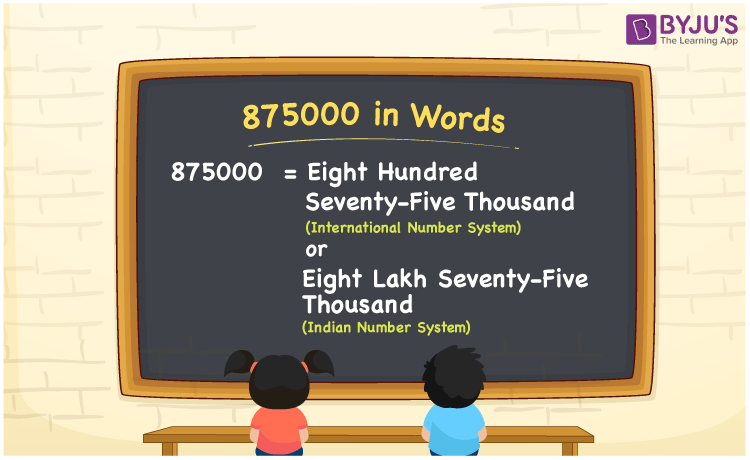# 875000 in Words

875000 in words is Eight hundred seventy-five thousand in the International number system and is Eight lakh seventy-five thousand in the Indian number system. As we can see, 875000 is a six-digit number, so we can write the word form of this number in different ways considering both International and Indian numeral systems. In this article, you will learn how to write the cardinal number 875000 in words.

 875000 in words Eight hundred seventy-five thousand (or) Eight lakh seventy-five thousand Eight hundred seventy-five thousand (or) Eight lakh seventy-five thousand in Numbers 875000

## 875000 in English Words

We generally express numbers in words using the English alphabet. Thus, we spell 875000 in English as “Eight lakh seventy-five thousand or Eight hundred seventy-five thousand”.## How to Write 875000 in Words?

875000 in words – International number system

The place value chart for 875000 with respect to the International number system is given below.

 Hundred thousands Ten thousands Thousands Hundreds Tens Ones 8 7 5 0 0 0

Here, ones = 0, tens = 0, hundreds = 0, thousands = 5, ten thousands = 2, hundred thousands = 8

These digits can be expanded as:

8 × 100 thousand + 7 × 10 thousand + 5 × thousand

= 800 thousand + 70 thousand + 5 thousand

= (800 + 70 + 5) thousand

= 875 thousand

Therefore, 875000 in words = 875 thousand = Eight hundred seventy-five thousand

875000 in words – Indian number system

Below is the place value chart for the number 875000 in the Indian system.

 Lakhs Ten thousands Thousands Hundreds Tens Ones 8 7 5 0 0 0

Here, ones = 0, tens = 0, hundreds = 0, thousands = 5, ten thousands = 7, lakhs = 8

The above digits can be expanded as follows.

= 8 × lakh + 7 × ten thousand + 5 × thousand + 0 × hundred + 0 × tens + 0 × ones

= Eight lakh + Seventy thousand + Five thousand

=Eight lakh seventy-five thousand

Therefore, 875000 in words = Eight lakh seventy-five thousand.

875000 is a natural number that precedes 875001 and succeeds 874999.

875000 in words – Eight lakh seventy-five thousand (or) Eight hundred seventy-five thousand

Is 875000 an odd number? – No

Is 875000 an even number? – Yes

Is 875000 a perfect square number? – No

Is 875000 a perfect cube number? – No

Is 875000 a prime number? – No

Is 875000 a composite number? – Yes

## Frequently Asked Questions on 875000 in Words

Q1

### How do you write 875000 in words?

We can write the number 875000 in words as Eight hundred seventy-five thousand or Eight lakh seventy-five thousand.
Q2

### How to write Rs. 875000 in words on a cheque?

On a cheque, we generally write Rs. 875000 in words as “Eight hundred seventy-five thousand rupees only or Eight lakh seventy-five thousand rupees only”.
Q3

### Express Eight lakh seventy-five thousand in numbers.

We can express Eight lakh seventy-five thousand in numbers as 875000.